Latest Banking jobs   »   Reasoning Ability Quiz For SBI Clerk...

# Reasoning Ability Quiz For SBI Clerk Prelims 2022- 01st May

Directions (1-5): Study the information carefully and answer the questions given below.
An uncertain number of persons sitting in a straight-line facing north. Three persons sit between P and L. M sits second to the right of P. Only three persons sit between L and R. S is immediate left of P. M sits fifth from one of the extreme ends of the row. As many persons sit between M and Q as many between Q and R. T sits second to the left of X who is an immediate neighbour of L. Not more than two persons sit between X and R. Five persons sit between R and Y. As many persons sit between R and Z as many between Z and Y.

Q1. How many persons sitting in a row?
(a) 20
(b) 18
(c) 23
(d) 14
(e) None of these

Q2. Who among the following person sits fourth to the right of Z?
(a) T
(b) X
(c) R
(d) L
(e) None of these

Q3. How many persons are sitting between X and M?
(a) Two
(b) Five
(c) Three
(d) Four
(e) More than five

Q4. If J is an immediate neighbour of S, then who among the following person sits second to the left of J?
(a) P
(b) X
(c) L
(d) Q
(e) None of these

Q5. Four of the following five are alike in a certain way based on a group, find the one that does not belong to that group?
(a) R-X
(b) Q-P
(c) M-S
(d) Z-T
(e) L-T

Directions (6-7): Read the given information carefully and answer the given question.
Point E is 10m to the east of Point G. Point H is 5m to the north of Point G. Point D is 5m to the west of Point H. Point C is 8m to the south of Point D. Point F is 11m to the east of Point C. Point K is 6m to the north of Point F.

Q6. How far and in which direction is Point K with respect to Point E?
(a) 5m south-east
(b) 8m, west
(c) 5m, north-west
(d) 8m, south-east
(e) 6m, south

Q7. If point K is 2m to the south of point P, then what is the distance between P and D?
(a) 11m
(b) 8m
(c) 15m
(d) 5m
(e) 9m

Direction (8-10): Study the following information carefully to answer the given questions:
In a family D is the son-in-law of W. U is the brother-in-law of E. S is the only child of E. J is married to U. Q is the granddaughter of W. L is the son of S. W is the wife of E. E has no sister.

Q8. How is S related to U?
(a) Mother
(b) Niece
(c) Uncle
(d) Brother
(e) None of these

Q9. How is L related to Q?
(a) Mother
(b) Sister
(c) Uncle
(d) Brother
(e) None of these

Q10. How is J related to W?
(a) Mother-in-law
(b) Sister-in-law
(c) Uncle
(d) Brother
(e) None of these

Q11. Aman is 21st from the left end of a row of 47 students and Monika is 23rd from the right end in the same row. How many students are there between them in the row?
(a) 1
(b) 3
(c) 2
(d) 5
(e) None of these

Directions (12-14): Study the following information carefully and answer the given questions.
Amongst five persons, P, R, S, T and U, each has different weight. P’s weight is more than R and T. S weight is less than U. Only two persons weight is more than P, whose weight is 65kg. Youngest person weight is 55kg.

Q12. Who among the following is youngest person?
(a) U
(b) R
(c) S
(d) T
(e) Can’t be determined

Q13. If U’s weight is 70kg, then what may be the weight of S?
(a) 63
(b) 64
(c) 68
(d) 72
(e) None of these

Q14. How many persons are heavier than R?
(a) One
(b) Two
(c) Three
(d) None
(e) Can’t be determined

Q15. Adarsh ranked 25th from the top and 36th from the bottom in a class. How many students are there in the class?
(a) 61
(b) 59
(c) 52
(d) 58
(e) 60

Solutions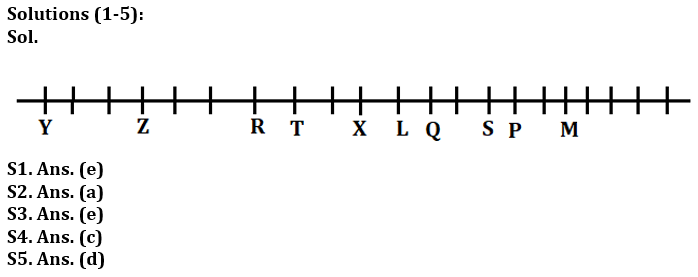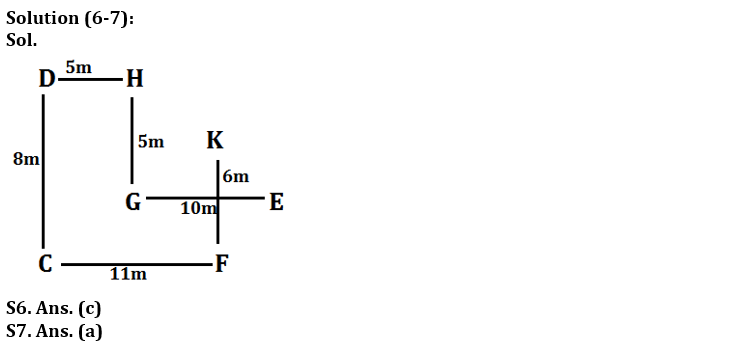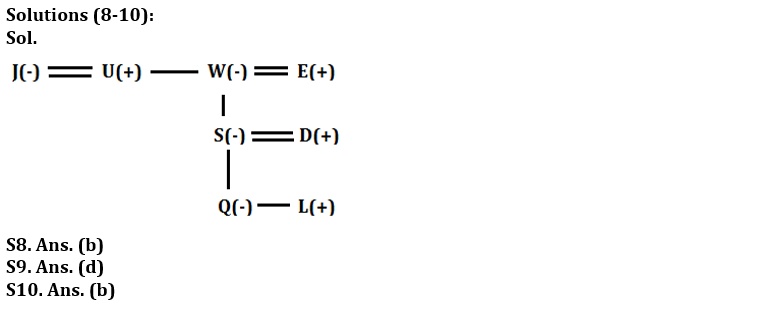S11. Ans. (b)
Sol. Aman position from right end = (47+1-21) = 27
Students between them= (27-23-1) = 3

S12. Ans. (e)
Sol. The arrangement will be: U > S > P > R / T > T / R

S13. Ans. (c)
Sol. The arrangement will be: U > S > P > R / T > T / R

S14. Ans. (e)
Sol. The arrangement will be: U > S > P > R / T > T / R

S15. Ans. (e)
Sol. Number of students in the class= 25+36-1=60#### Congratulations!

Incorrect details? Fill the form again here

•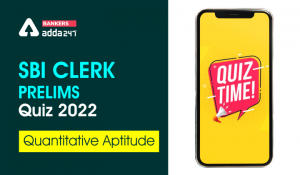Quantitative Aptitude Quiz For SBI Clerk...
•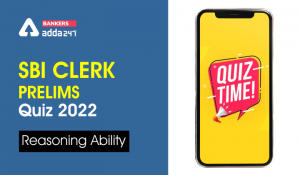Reasoning Ability Quiz For SBI Clerk Pre...
•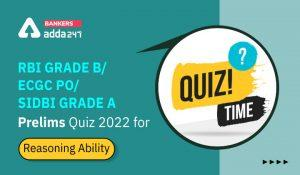Reasoning Ability Quiz For RBI Grade B/ ...
•Reasoning Ability Quiz For SBI Clerk Pre...
•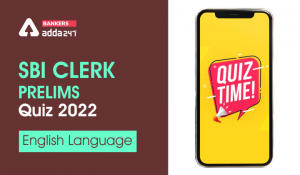English Quizzes For SBI Clerk Prelims 20...
•Reasoning Ability Quiz For RBI Grade B/ ...